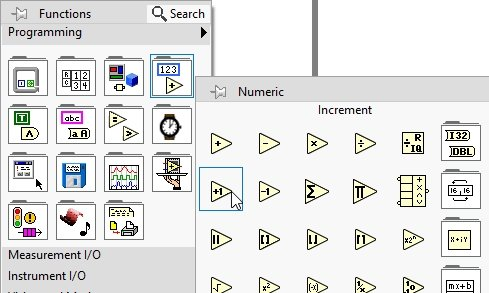# Sum of N numbers project in labview: tutorial 34

In this tutorial I will help you to design a VI that will take a number as input from the user and at the output it will return the sum of natural numbers up to that number. For example, the user enters 3 as an input value to the VI then the output of the VI will return 1+2+3 = 6 in the indicator. We will be using a ‘while’ loop to do so. At the end of the tutorial you are provided with an exercise to do it by yourself, and in the next tutorials I will assume that you have done those exercises and I will not explain the concept regarding them.

Explanation using a VI:

Figure 1: Numeric control placement

Figure 2: While loop placement

Figure 4: Creating numeric constant

Figure 5: Shift register constant

• At one of the two inputs of the add block connect the right shift register, and the output of this block connect the right shift register.
• The purpose of this will be, the right shift register will store the value of sum from the previous iteration and will pass it on to the left shift register in the next iteration, as we have discussed in detail in the tutorial related to shift registers.
• Now on the other input of the add block we have to connect the incremented iteration because the number of iterations start from 0 but we want sum from 1 onwards. From the function palette select numeric and then select increment as shown in the figure below,Figure 7: Increment block placement

Figure 9: Greater than or equal block

Figure 10: Termination condition

Figure 11: Output indicator

Figure 12: Complete block diagram

Figure 13: Output 1

Figure 14: Output 2

Exercise:

• Design a VI that will take two input integers and calculate the sum of natural numbers between those two integers

<<Previous tutorial                                       Next tutorial>>